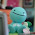## Sunday, January 16, 2011

### Final Percent Post

Definition of percent:
any number out of 100,that can be represented as a fraction and a decimal.

Key Points of Chapter 4

4.1

When showing a percent greater than 100%, use more than 1 grid.
When showing a percent less than 1%, but more than 0%, use only one grid.

4.2

Percents can be shown as a decimal and fraction.
50% = 1/2 and 0.5

4.3
To calculate a percent of a number,write it as a decimal and multiply it.
15 1/2% of 50= 0.125x50
= 6.25

4.4
To combine a percent, put them,or add them together.
ex.1% + 2% = 3%
To found out the increase in a number, add the combined percent to the original number.
5% + 7% = 12%

1.